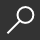## Solitons, Instantons, and Twistors by Maciej Dunajski

Overview -
Most nonlinear differential equations arising in natural sciences admit chaotic behavior and cannot be solved analytically. Integrable systems lie on the other extreme. They possess regular, stable, and well behaved solutions known as solitons and instantons. These solutions play important
roles in pure and applied mathematics as well as in theoretical physics where they describe configurations topologically different from vacuum. While integrable equations in lower space-time dimensions can be solved using the inverse scattering transform, the higher-dimensional examples of
anti-self-dual Yang-Mills and Einstein equations require twistor theory. Both techniques rely on an ability to represent nonlinear equations as compatibility conditions for overdetermined systems of linear differential equations.

The book provides a self-contained and accessible introduction to the subject. It starts with an introduction to integrability of ordinary and partial differential equations. Subsequent chapters explore symmetry analysis, gauge theory, gravitational instantons, twistor transforms, and
anti-self-duality equations. The three appendices cover basic differential geometry, complex manifold theory, and the exterior differential system.

local_shippingFor DeliveryOn Order. Usually ships in 2-4 weeks
This item is Non-Returnable.
FREE Shipping for Club Members helpNew & Used Marketplace 4 copies from \$112.00

## More About Solitons, Instantons, and Twistors by Maciej Dunajski

### Overview

Most nonlinear differential equations arising in natural sciences admit chaotic behavior and cannot be solved analytically. Integrable systems lie on the other extreme. They possess regular, stable, and well behaved solutions known as solitons and instantons. These solutions play important
roles in pure and applied mathematics as well as in theoretical physics where they describe configurations topologically different from vacuum. While integrable equations in lower space-time dimensions can be solved using the inverse scattering transform, the higher-dimensional examples of
anti-self-dual Yang-Mills and Einstein equations require twistor theory. Both techniques rely on an ability to represent nonlinear equations as compatibility conditions for overdetermined systems of linear differential equations.

The book provides a self-contained and accessible introduction to the subject. It starts with an introduction to integrability of ordinary and partial differential equations. Subsequent chapters explore symmetry analysis, gauge theory, gravitational instantons, twistor transforms, and
anti-self-duality equations. The three appendices cover basic differential geometry, complex manifold theory, and the exterior differential system.

This item is Non-Returnable.

### Details

• ISBN-13: 9780198570639
• ISBN-10: 0198570635
• Publisher: Oxford University Press, USA
• Publish Date: February 2010
• Page Count: 368
• Dimensions: 9.1 x 6.1 x 0.9 inches
• Shipping Weight: 1.27 poundsRelated Categories